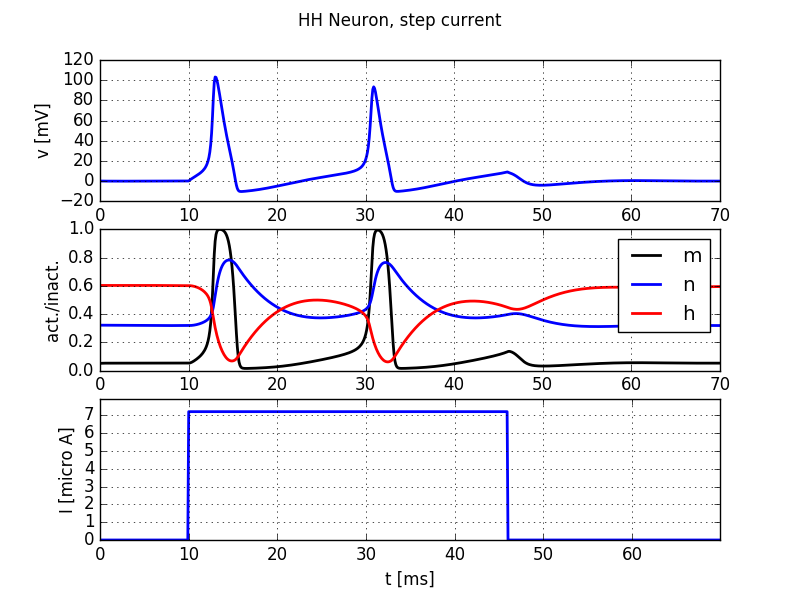# 5. Numerical integration of the HH model of the squid axon¶

Book chapters

See Chapter 2 Section 2 on general information about the Hodgkin-Huxley equations and models.

Python classes

The hodgkin_huxley.HH module contains all code required for this exercise. It implements a Hodgkin-Huxley neuron model. At the beginning of your exercise solutions, import the modules and run the demo function.

%matplotlib inline
import brian2 as b2
import matplotlib.pyplot as plt
import numpy as np
from neurodynex3.hodgkin_huxley import HH
from neurodynex3.tools import input_factory

HH.getting_started()Step current injection into a Hodgkin-Huxley neuron

## 5.1. Exercise: step current response¶

We study the response of a Hodgkin-Huxley neuron to different input currents. Have a look at the documentation of the functions HH.simulate_HH_neuron() and HH.plot_data() and the module neurodynex3.tools.input_factory.

### 5.1.1. Question¶

What is the lowest step current amplitude $$I_{min}$$ for generating at least one spike? Determine the value by trying different input amplitudes in the code fragment:

current = input_factory.get_step_current(5, 100, b2.ms, I_min *b2.uA)
state_monitor = HH.simulate_HH_neuron(current, 120 * b2.ms)
HH.plot_data(state_monitor, title="HH Neuron, minimal current")


### 5.1.2. Question¶

• What is the lowest step current amplitude to generate repetitive firing?
• Discuss the difference between the two regimes.

## 5.2. Exercise: slow and fast ramp current¶

The minimal current to elicit a spike does not just depend on the amplitude $$I$$ or on the total charge $$Q$$ of the current, but on the “shape” of the current. Let’s see why:

### 5.2.1. Question¶

Inject a slow ramp current into a HH neuron. The current has amplitude 0A at t in [0, 5] ms and linearly increases to an amplitude of 12.0uAmp at t=ramp_t_end. At t>ramp_t_end, the current is set to 0A. Using the following code, reduce slow_ramp_t_end to the maximal duration of the ramp current, such that the neuron does not spike. Make sure you simulate system for at least 20ms after the current stops.

• What is the membrane voltage at the time when the current injection stops (t=slow_ramp_t_end)?
b2.defaultclock.dt = 0.02*b2.ms
slow_ramp_t_end = 60  # no spike. make it shorter
slow_ramp_current = input_factory.get_ramp_current(5, slow_ramp_t_end, b2.ms, 0.*b2.uA, 12.0*b2.uA)
state_monitor = HH.simulate_HH_neuron(slow_ramp_current, 90 * b2.ms)
idx_t_end = int(round(slow_ramp_t_end*b2.ms / b2.defaultclock.dt))
voltage_slow = state_monitor.vm[0,idx_t_end]
print("voltage_slow={}".format(voltage_slow))


### 5.2.2. Question¶

Do the same as before but for a fast ramp current: The maximal amplitude at t=ramp_t_end is 4.5uAmp. Start with fast_ramp_t_end = 8ms and then increase it until you observe a spike. Note: Technically the input current is implemented using a TimedArray. For a short, steep ramp, the one millisecond discretization for the current is not high enough. You can create a finer resolution by setting the parameter unit_time in the function input_factory.get_ramp_current() (see next code block).

• What is the membrane voltage at the time when the current injection stops (t=fast_ramp_t_end)?
b2.defaultclock.dt = 0.02*b2.ms
fast_ramp_t_end = 80  # no spike. make it longer
fast_ramp_current = input_factory.get_ramp_current(50, fast_ramp_t_end, 0.1*b2.ms, 0.*b2.uA, 4.5*b2.uA)
state_monitor = HH.simulate_HH_neuron(fast_ramp_current, 40 * b2.ms)
idx_t_end = int(round(fast_ramp_t_end*0.1*b2.ms / b2.defaultclock.dt))
voltage_fast = state_monitor.vm[0,idx_t_end]
print("voltage_fast={}".format(voltage_fast))


### 5.2.3. Question¶

Use the function HH.plot_data() to visualize the dynamics of the system for the fast and the slow case above. Discuss the differences between the two situations. Why are the two “threshold” voltages different? Link your observation to the gating variables $$m$$, $$n$$, and $$h$$. Hint: have a look at Chapter 2 Figure 2.3.

## 5.3. Exercise: Rebound Spike¶

A HH neuron can spike not only if it receives a sufficiently strong depolarizing input current but also after a hyperpolarizing current. Such a spike is called a rebound spike.

### 5.3.1. Question¶

Inject a hyperpolarizing step current I_amp = -1 uA for 20ms into the HH neuron. Simulate the neuron for 50 ms and plot the voltage trace and the gating variables. Repeat the simulation with I_amp = -5 uA What is happening here? To which gating variable do you attribute this rebound spike?

## 5.4. Exercise: Brian implementation of a HH neuron¶

In this exercise you will learn to work with the Brian2 model equations. To do so, get the source code of the function HH.simulate_HH_neuron() (follow the link to the documentation and then click on the [source] link). Copy the function code and paste it into your Jupyter Notebook. Change the function name from simulate_HH_neuron to a name of your choice. Have a look at the source code and find the conductance parameters gK and gNa.

### 5.4.1. Question¶

In the source code of your function, change the density of sodium channels. Increase it by a factor of 1.4. Stimulate this modified neuron with a step current.

• What is the minimal current leading to repetitive spiking? Explain.
• Run a simulation with no input current to determine the resting potential of the neuron. Link your observation to the Goldman–Hodgkin–Katz voltage equation.
• If you increase the sodium conductance further, you can observe repetitive firing even in the absence of input, why?